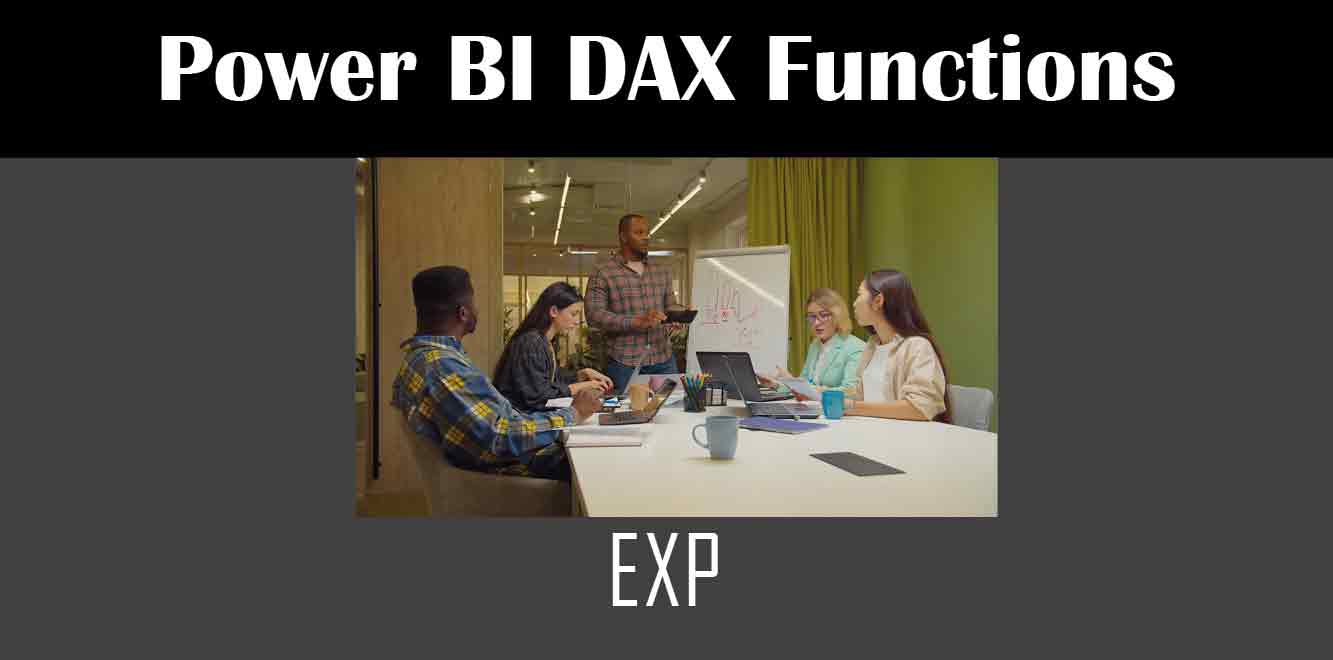# Exp

## Y## What is the Exp function?

The Exp function is a mathematical function that is used to calculate the exponential value of a number. The exponential value is calculated as e raised to the power of the given number. The value of e is approximately 2.71828. The syntax for the Exp function is as follows:

``` Exp(number) ```

Where `number` is the number for which we want to calculate the exponential value.

## How to use the Exp function in Power Apps

The Exp function can be used in Power Apps to calculate the exponential value of a number. Let’s take an example where we want to calculate the exponential value of a number entered by the user. We can create a text input control in our app and use the following formula in the Default property of the control:

``` Exp(Value(TextInput1.Text)) ```

In this formula, `TextInput1` is the name of our text input control. We are using the Value function to get the value entered by the user in the text input control and passing it to the Exp function to calculate the exponential value. The result will be displayed in the text input control.

We can also use the Exp function in a label control to display the exponential value of a number. Let’s take another example where we want to display the exponential value of a number entered by the user in a label control. We can use the following formula in the Text property of the label control:

``` "Exponential value of " & TextInput1.Text & " is " & Exp(Value(TextInput1.Text)) ```

In this formula, we are concatenating a string “Exponential value of ” with the value entered in the text input control and the calculated exponential value using the & operator. The result will be displayed in the label control.

The Exp function is a useful mathematical function in Power Apps that can be used to calculate the exponential value of a number. It is a simple and intuitive function that can be used in various scenarios. In this article, we discussed what the Exp function is and how to use it in Power Apps. We hope this article has been helpful in understanding the Exp function and its usage in Power Apps.